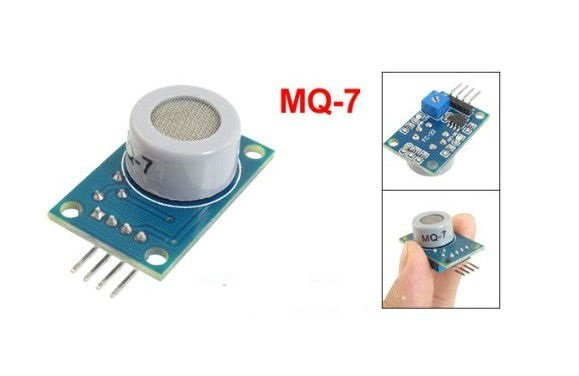# Chipkit and a MQ7 Gas SensorThe MQ7 Gas Sensor module is useful for gas leakage detection. They are used in gas leakage detecting equipments in home and industry, are suitable for detecting of Carbon Monoxide.  Due to its high sensitivity and response time, measurements can be taken as soon as possible.

The MQ-7 can detect Carbon Monoxide concentrations anywhere from 20 to 2000ppm.

The sensitivity of the sensor can be adjusted by using the potentiometer.FEATURES

* High sensitivity to Carbon monoxide
* Fast response .
* Stable and long life
* Simple drive circuit

Connection

here are the connections for my sensor

Wemos Gas Sensor
3v3 VCC
GND GND
NC DO
Analog A0 AO

Code

 Source code```void setup()
{
Serial.begin(9600);
}

void loop()
{
float sensorVoltage;
float sensorValue;

sensorVoltage = sensorValue/1024*3.3;

Serial.print("sensor voltage = ");
Serial.print(sensorVoltage);
Serial.println(" V");
delay(1000);
}```

Results

Again look at the serial monitor, you need to let the sensor stabilise. Remember it consists of a heating element, so you effectively have to let the sensor ‘heat up’.

sensor voltage = 1.54 V
sensor voltage = 1.51 V
sensor voltage = 1.50 V
sensor voltage = 1.47 V
sensor voltage = 1.46 V
sensor voltage = 1.44 V
sensor voltage = 1.43 V
sensor voltage = 1.41 V
sensor voltage = 1.39 V
sensor voltage = 1.38 V
sensor voltage = 0.71 V
sensor voltage = 0.71 V
sensor voltage = 0.71 V
sensor voltage = 0.71 V
sensor voltage = 0.71 V
sensor voltage = 0.71 V
sensor voltage = 0.70 V
sensor voltage = 0.70 V
sensor voltage = 0.71 V
sensor voltage = 0.70 V